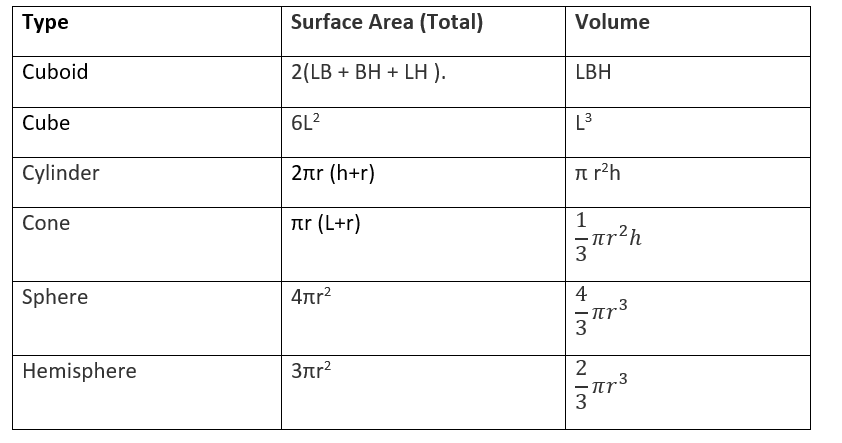# Class 9 Maths notes for Surface Area and Volume

Welcome to Class 9 Maths chapter 13 Surface Area and Volume notes.The topics in this page are Surface area,Volume,supplimentary angles, Surface Area and Volume of Cube,cuboid, Cylinder,cone,Sphere,Hemissphere along with examples, and questions and answers .This is according to CBSE and the NCERT textbook. If you like the study material, feel free to share the link as much as possible.

Table of Content

## Plane Figure

Plane figure are the figure which lies in a plane or to put it simply which we can draw on a piece of paper
We have already studied about perimeter and area of the plane figure
Just a recall of them

## Solid Figure

Solid figure does not lie in a single plane.They are three dimensional figure
Example:Cube ,Cylinder, Sphere

## Mensuration

• It is branch of mathematics which is concerned about the measurement of length ,area and Volume of plane and Solid figure

## Perimeter

• The perimeter of plane figure is defined as the length of the boundary
• It units is same as that of length i.e. m ,cm,km
 1 Meter 10 Decimeter 100 centimeter 1 Decimeter 10 centimeter 100 millimeter 1 Km 10 Hectometer 100 Decameter 1 Decameter 10 meter 1000 centimeter

## Surface Area or Area

• The area of the plane figure is the surface enclosed by its boundary
• It unit is square of length unit. i.e. m2 ,  km2
 1 square Meter 100 square Decimeter 10000 square centimeter 1 square Decimeter 100 square centimeter 10000 square millimeter 1 Hectare 100 squareDecameter 10000 square meter 1 square myraimeter 100 square kilometer 108  squaremeter

## Volume

 1 cm3 1mL 1000 mm3 1 Litre 1000mL 1000 cm3 1 m3 106  cm3 1000 L 1 dm3 1000 cm3 1 L

## Surface Area and Volume of Cube and Cuboid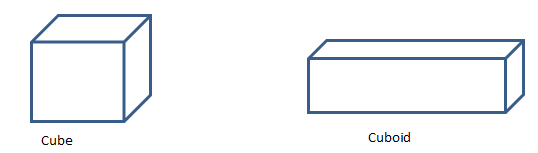Type Measurement Surface Area of Cuboid of Length L, Breadth B and Height H $2( LB + BH + LH )$. Lateral surface area of the cuboids $2( L + B ) \times H$ Diagonal of the cuboids $\sqrt {L^2+B^2+H^2}$ Volume of a cuboids $L \times B \times H$ Length of all 12 edges of the cuboids $4 \times (L+B+H)$. Surface Area of Cube of side L $6L^2$ Lateral surface area of the cube $4L^2$ Diagonal of the cube $L \sqrt {3}$ Volume of a cube $L^3$

## Surface Area and Volume of Right circular cylinder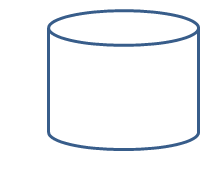Radius The radius (r) of the circular base is called the radius of the cylinder Height The length of the axis of the cylinder is called the height (h) of the cylinder Lateral Surface The curved surface joining the two base of a right circular cylinder is called Lateral Surface.
 Type Measurement Curved or lateral Surface Area of cylinder $2 \pi rh$ Total surface area of cylinder $2 \pi r (h+r)$ Volume of Cylinder $\pi r^2 h$

## Surface Area and Volume of Right circular cone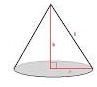Radius The radius (r) of the circular base is called the radius of the cone Height The length of the line segment joining the vertex to the centre of base is called the height (h) of the cone. Slant Height The length of the segment joining the vertex to any point on the circular edge of the base is called the slant height (L) of the cone. Lateral surface Area The curved surface joining the  base and uppermost point of a right circular cone is called Lateral Surface
 Type Measurement Curved or lateral Surface Area of cone $\pi rL$ Total surface area of cone $\pi r (L+r)$ Volume of Cone $\frac {1}{3} \pi r^2 h$

## Surface Area and Volume of sphere and hemisphere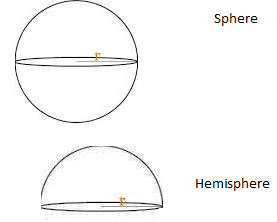Sphere A sphere can also be considered as a solid obtained on rotating a circle About its diameter Hemisphere A plane through the centre of the sphere divides the sphere into two equal parts, each of which is called a hemisphere radius The radius of the circle by which it is formed Spherical Shell The difference of two solid concentric spheres is called a spherical shell Lateral Surface Area  for Sphere Total surface area of the sphere Lateral Surface area of Hemisphere It is the curved surface area leaving the circular base
 Type Measurement Surface area of Sphere $4 \pi r^2$ Volume of Sphere $\frac {4}{3} \pi r^3$ Curved Surface area of hemisphere $2 \pi r^2$ Total Surface area of hemisphere $3 \pi r^2$ Volume of hemisphere $\frac {2}{3} \pi r^3$ Volume of the spherical shell whose outer and inner radii and ‘R’ and ‘r’ respectively $\frac {2}{3} \pi (R^3-r^3)$

## How the Surface area and Volume are determined

 Area of Circle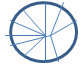The circumference of a circle is $2 \pi r$. This is the definition of π (pi). Divide the circle into many triangular segments. The area of the triangles is 1/2 times the sum of their bases, 2πr (the circumference), times their height, r. $A= \frac {1}{2} \times 2 \pi r \times r= \pi r^2$ Surface Area of cylinder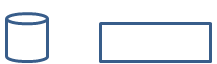This can be imagined as unwrapping the surface into a rectangle. Surface area of cone This can be achieved by divide the surface of the cone  into its triangles, or the surface of the cone into many thin triangles. The area of the triangles is 1/2 times the sum of their bases, p, times their height, $A= \frac {1}{2} \times 2 \pi r \times s=\pi rs$

## How to solve Surface Area and Volume Problem

(1) We have told explained the surface area and volume of various common sold shapes.
(2) Try to divide the given solid shape into known shapes if the solid figure is other than known shapes
(3) Find out the given quantities like radius,height
(4) Apply the formula from the above given tables and get the answer
(5) Make sure you use common units across the problem

## Solved example

Question 1
A cylinder is 50 cm in diameter and 3.5 m in height
(b) Find the Curved Surface area
(c) Total Surface area
(d) Volume
Solution
Height of the cylindrical pillar =h=3.5 m
Diameter of the cylindrical pillar =d=50 cm
So Radius of the cylindrical pillar =r=50/2=25 cm =0.25 m
So Curved surface of the cylindrical pillar =$2 \pi \times r \times h=5.5$ m2
Total Surface area= $2 \pi r h + 2 \pi r^2 =5.89$ m2
Volume =$\pi r^2 h=.17$ m3

Question 2
A cubical box has each edge 10 cm and another cuboidal box is 12.5 cm long, 10 cm wide and 8 cm high.
(i) Which box has the greater lateral surface area and by how much?
(ii) Which box has the smaller total surface area and by how much?
Solution:
(i)
Dimension of Cube
Edge of cube (a) = 10 cm
Dimension of Cuboidal
Length (l) of box = 12.5 cm
Breadth (b) of box = 10 cm
Height (h) of box = 8 cm
Lateral surface area of cubical box  is given by  4(a)2
= 4(10 cm)2
= 400 cm2
Lateral surface area of cuboidal box  is given by  2[lh + bh]
= [2(12.5 × 8 + 10 × 8)] cm2
= (2 × 180) cm2
= 360 cm2
It is apparent from the data, 400 > 360
The difference =Lateral surface area of cubical box − Lateral surface area of cuboidal box = 400 cm2 − 360 cm2 = 40 cm2
So Lateral surface area of cubical box is greater than Lateral surface area of cuboidal box by 40 cm2
(ii) Total surface area of cubical box = 6(a)2 = 6(10 cm)2 = 600 cm2
Total surface area of cuboidal box
= 2[lh + bh + lb]
= [2(12.5 × 8 + 10 × 8 + 12.5 × 10] cm2
= 610 cm2
It is apparent from the data, 610 > 600
Difference=Total surface area of cuboidal box − Total surface area of cubical box = 610 cm2 − 600 cm2 = 10 cm2
So Lateral surface area of cuboidal box is greater than Lateral surface area of cubical box by 10 cm2

## Summary

Here is surface area and volume class 9 notes Summary